# GSEB Solutions Class 10 Maths Chapter 3 Pair of Linear Equations in Two Variables Ex 3.5

Gujarat Board GSEB Solutions Class 10 Maths Chapter 3 Pair of Linear Equations in Two Variables Ex 3.5 Textbook Questions and Answers.

## Gujarat Board Textbook Solutions Class 10 Maths Chapter 3 Pair of Linear Equations in Two Variables Ex 3.5

Question 1.
Which of the following pairs of linear equations has unique solution, no solution, or infinitely many solutions. In case there is a unique solution, find it by using cross multiplication method.

1. x – 3y – 3 = 0, 3x – 9y – 2 = 0
2. 2x + y = 5, 3x + 2y = 8
3. 3x – 5y = 20, 6x – 10y = 40
4. x – 3y – 7 = 0, 3x – 3y – 15 = 0

Solution:
1. The given pair of linear equations is
x – 3y – 3 = 0 …(1)
3x – 9y – 2 = 0 …(2)
Here, a1 = 1, b1 = -3, c1 = -3
a2 = 3, b2 = -9, c2 = -2
We see that
$$\frac{a_{1}}{a_{2}}=\frac{b_{1}}{b_{2}} \neq \frac{c_{1}}{c_{2}}$$
Hence, the given pair of linear equations has no solution.2. The given pair of linear equations is
2x + y = 5
3x + 2y = 8
⇒ 2x +y – 5 = 0
3x + 2y – 8 = 0
Here, a1 = 2, b1 = 1, c1 = -5
a2 = – 3, b2 = 2, c2 = -8
We see that
$$\frac{a_{1}}{a_{2}} \neq \frac{b_{1}}{b_{2}}$$
Hence, the given pair of linear equations has a unique solution.
To solve the given equation by cross multiplication method, we draw the diagram below:Hence, the required solution of the given pair of linear equations is x = 2, y = 1.
Verification:
Substituting x = 2, y = 1, we find that both the equations (1) and (2) are satisfied as shown below:
2x + y = 2(2) + 1
= 4 + 1 = 5
3x + 2y = 3(2) + 2(1)
= 6 + 2 = 8
Hence, the solution is correct.3. The given pair of linear equations is
3x – 5y = 20 …(1)
6x – 10y = 40 …(2)
⇒ 3x – 5y – 20 = 0
6x – 10y – 40 = 0
Here, a1 = 3, b1 = -5, c1 = -20
a2 = 6, b2 = -10, c2 = -40
We see that
$$\frac{a_{1}}{a_{2}}=\frac{b_{1}}{b_{2}}=\frac{c_{1}}{c_{2}}$$
Hence, the given pair of linear equations has infinitely many solutions.

4. The given pair of linear equations is
x – 3y – 7 = 0 …(1)
3x – 3y – 15 = 0 …(2)
Here, a1 = 1 , b1 = -3, c1 = -7
a2 = 3, b2 = 3, c2 = -15
We see that
$$\frac{a_{1}}{a_{2}} \neq \frac{b_{1}}{b_{2}}$$
Hence, the given pair of linear equations has a unique solution.
To solve the given equation by cross multiplication method, we draw the diagram below:Hence, the required solution of the given pair of linear equations is x = 4, y = -1.
Verification:
Substituting x – 4, y = 1, we find that both the equations (1) and (2) are satisfied as shown below:
x – 3y – 7 = 4 – 3(-1) – 7 = 0
3x – 3y – 15 = 3(4) – 3(-1) – 15 = 0
Hence, the solution is correct.Question 2.
1. For which values of a and b does the following pair of linear equations have an infinite number of solutions?
2x + 3y = 7
(a – b)x + (a + b)y = 3a + b – 2
2. For which value of k will the following pair of linear equations have no solution?
3x + y = 1
(2k – 1)x + (k – 1)y = 2k + 1
Solution:
1. The given pair of linear equations is 2x + 3y = 7
(a – b)x + (a + b)y = 3a + b – 2
Here, a1 = 2, b1 = 3, c1 = 7
a2 = a – b, b2 = a + b, c2 = 3a + b – 2
Since, the given pair of linear equations has infinite solution,From first two,
$$\frac{2}{a-b}=\frac{3}{a+b}$$
⇒ 2(a + b) = 3(a – b)
⇒ 2a + 26 = 3a – 3b
⇒ a – 5b = 0 ……(1)For last two,
$$\frac{3}{a+b}=\frac{7}{3 a+b-2}$$
⇒ 3(3a + b – 2) = 7(a + b)
⇒ 9a + 3b – b = 7a + 7b
⇒ 2a – 4b – b = 0
⇒ a – 2b – 3 = 0 …(2)
To solve the equations (1) and (2) by cross-multiplication method, we draw the diagram below: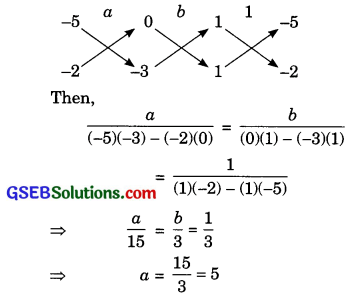and b = $$\frac{3}{3}$$ = 1
Hence, the required values of a and b are 5 and 1 respectively.

2. The given pair of linear equations is:
3x + y = 1
(2k – 1)x + (k – 1)y = 2k + 1
⇒ 3x + y – 1 = 0
(2k – 1)x + (k – 1 )y -(2k + 1) = 0
Here, a1 = 3, b1 = 1, c1 = -1
a2 = 2k – 1, b2 = k – 1, c2 = -(2k + 1)
For having no solution, we must have⇒ 3(k – 1) = 2k – 1
⇒ 3k – 3 = 2k – 1
⇒ 3k – 2k = 3 – 1
⇒ k = 2
Hence, the required value of k is 2.Question 3.
Solve the following pair of linear equations by the substitution and cross-multiplication methods.
8x + 5y = 9
3x + 2y = 4
Solution:
The given pair of linear equations is
8x + 5y = 9 …(1)
3ox + 2y = 4 …(2)
I. By Substitution method:
From equation (2),
2y = 4 – 3x
⇒ y = $$\frac{4-3 x}{2}$$ ……(3)
Substitute this value of y in equation (1), we get
8x + 5$$\left(\frac{4-3 x}{2}\right)$$ = 9
⇒ 16x + 20 – 15x = 18
⇒ x + 20 = 18
⇒ x = 18 – 20
⇒ x = – 2
Substituting this value of x in equation (3), we get
$$y=\frac{4-3(-2)}{2}$$
$$=\frac{4+6}{2}=\frac{10}{2}=5$$
So, the solution of the given pair of linear equations is x = -2, y = 5.II. By Cross-multiplication method:
Let us write the given pair of linear equations is
8x + 5y – 9 = 0 …(3)
3x + 2y – 4 = 0 …(4)
To solve the equations (3) and (4) by cross-multiplication method, we draw the diagram below: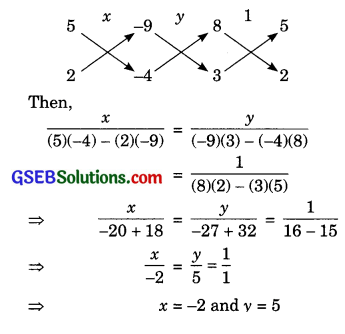Hence, the required solution of the given pair of linear equations is
x = -2, y = 5.
Verification:
Substituting x = -2, y = 5, we find that both the equations (1) and (2) are satisfied as shown below:
8x + 5y = 8(-2) + 5(5)
= -16 + 25 = 9
3x + 2y = 3(-2) + 2(5)
= -6 + 10 = 4
Hence, the solution is correct.Question 4.
Form the pair of linear equations in the following problems and find their solutions (if they exist) by any algebraic method:

(i) A part of monthly hostel charges is fixed and the remaining depends on the number of days one has taken food in the mess. When a student A takes food for 20 days she has to pay ₹ 1000 as hostel charges whereas a student B, who takes food for 26 days, pays ₹ 1180 as hostel charges. Find the fixed charge and the cost of food per day.

(ii) A fraction becomes $$\frac{1}{3}$$ when 1 is subtracted from the numerator and it becomes $$\frac{1}{4}$$ when 8 is added to its denominator. Find the fraction.

(iii) Yash scored 40 marks in a test, getting 3 marks for each right answer and losing 1 mark for each wrong answer. Had 4 marks been awarded for each correct answer and 2 marks been deducted for each incorrect answer, then Yash would have scored 50 marks. How many questions were there in the test?

(iv) Places A and B are 100 km apart on a highway. One car starts from A and another from B at the same time. If the cars travel in the same direction at different speeds, they meet in 5 hours. If they travel towards each other they meet in 1 hour. What are the speeds of the two cars?

(v) The area of a rectangle gets reduced by 9 square units if its length is reduced by 5 units and breadth is increased by 3 units. If we increase the length by 3 units and the breadth by 2 units, the area increases by 67 square units. Find the dimensions of the rectangle.

Solution:
(i) Let the fixed charge be ₹ x and the cost of food per day be ₹ y.
Then according to the question,
x + 20y = 1000 …(1)
x + 26y = 1180 …(2)
⇒ x + 20y – 1000 = 0 …(3)
x + 26y – 1180 = 0 …(4)
To solve the equations (3) and (4) by cross-multiplication method, we draw the diagonal below: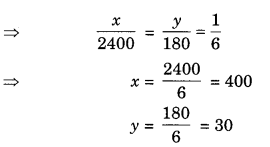Hence, the fixed charge is ₹ 400 and the cost of food per day is ₹ 30.
Verification:
Substituting x = 400, y = 30, we find out both the equations (1) and (2) are satisfied as shown below:
x + 20y = 400 + 20(30)
= 400 + 600 = 1000
x + 26y = 400 + 26(30)
= 400 + 780
= 1180
Hence, the solution is correct.(ii) Let the fraction be $$\frac{x}{y}$$.
Then, according to the question,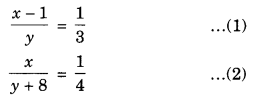3(x – 1) = y
4x = y + 8 …….(3)
⇒ 3x – y – 3 = 0
4x – y – 8 = 0
To solve the equations (3) and (4) by cross-multiplication method, we draw the diagram below: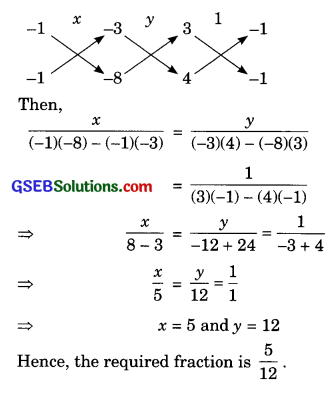Verification:
Substituting x = 5, y = 12, we find that both the equations (1) and (2) are satisfied as shown below:Hence, the solution is correct.(iii) Suppose that Yash gave right answers to x questions and wrong answers toy questions. Then, the total number of questions in the test = x + y.
Also, according to the question,
3x – y = 40 ……(1)
4x – 2y = 50 …….(2)
⇒ 3x – y – 40 = 0 ……(3)
4x – 2y – 50 = 0 ……(4)
To solve the equations (3) and (4) by cross-multiplication method, we draw the diagram below: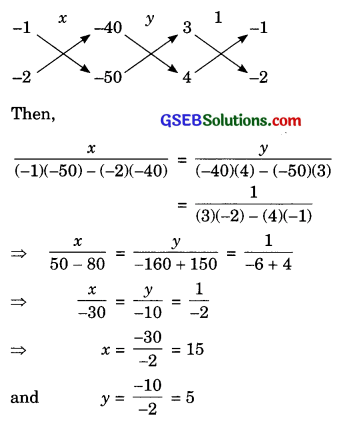Hence, Yash gave right answers to 15 questions and wrong answers to 5 questions. Therefore, total number of questions in the test = x + y = 15 + 5 = 20.
Verification:
Substituting x = 15, y = 5, we find that both the equations (1) and (2) are satisfied as shown below:
3x – y = 3(15) – 5
= 45 – 5 = 40
4x – 2y = 4(15) – 2 × (15)
= 60 – 10 = 50
Hence, the solution is correct.(iv) Let the speeds of two cars be x km/hour and y km/hour respectively.
Case I:
When the cars travel in the same direction.
Let they meet at P.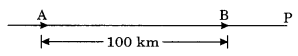Distance travelled by the car starting from A in 5 hours = AP = 5x km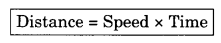Distance travelled by the car starting from B in 5 hours = BP = 5y km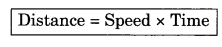Now,
AP – BP = 100
5x – 5y = 100
x – y = 20 …(1)

Case II:
When the cars travel towards each other.
Let they meet at Q.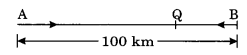Distance travelled by the car starting from A in 1 hour = AQ = x km
Distance travelled by the car starting from B in 1 hour = BQ = y km
Now, AQ + BQ = 100
x + y = 100 ……(2)
Equation (1) and (2) can be re-written as
x – y – 20 = 0 …(3)
x + y – 100 = 0 …(4)
To solve the equations (3) and (4) by cross-multiplication method, we draw the diagram below: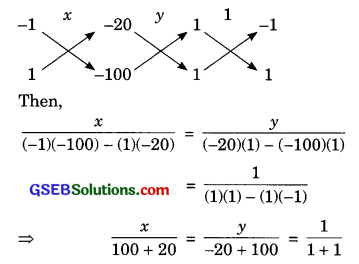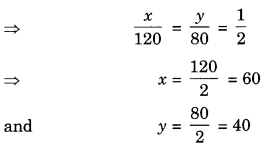Hence, the speeds of the two cars are 60 km/hour and 40 km/hour respectively.
Verification:
Substituting x = 60, y = 40, we find that both the equations (1) and (2) are satisfied as shown below
x – y – 60 – 40 = 20
x + y = 60 + 40 = 100
Hence, the solution is correct.(v) Let the dimensions (i.e., the length and the breadth) of the rectangle be x units and y units respectively.
Then, area of the rectangle
= xy square units
According to the question,
xy – 9 = (x – 5)(y + 3)
⇒ xy – 9 = xy + 3x – 5y – 15
⇒ 3x – 5y – 6 = 0 …(1)
and xy + 67 = (x + 3)(y + 2)
⇒ xy + 67 = xy + 2x + 3y + 6
⇒ 2x + 3y – 61 = 0 …(2)
To solve the equations (1) and (2) by cross-multiplication method, we draw the diagram below: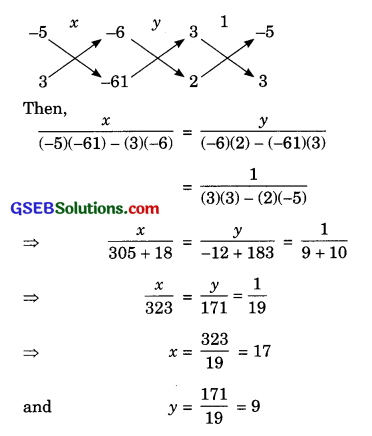Hence, the dimensions (i.e., the length and the breadth) of the rectangle are 17 units and 9 units respectively.
Verification:
Substituting x = 17, y = 9, we find that both the equations (1) and (2) are satisfied as shown below:
3x-5y – 6 = 3(17) – 5(9) – 6
= 51 – 45 – 6 = 0
2x + 3y – 61 = 2(17) + 3(9) – 61
= 34 + 27 – 61 = 0
Hence, the solution is correct.